# Tangent Circle Formula

In geometry, a tangent of a circle is a straight line that touches the circle at exactly one point, never entering the circle’s interior. It is a line through a pair of infinitely close points on the circle.

The tangent lines to circles form the subject of several theorems and play an important role in many geometrical constructions and proofs. Since the tangent line to a circle at a point P is perpendicular to the radius to that point, theorems involving tangent lines often involve radial lines and orthogonal circles.

The tangent has two defining properties such as:

• A Tangent touches a circle in exactly one place.
• The Tangent intersects the circle’s radius at
$$\begin{array}{l}90^{\circ}\end{array}$$
angle.

The equation of the tangent is written as,

$$\begin{array}{l}\huge \left(y-y_{0}\right)=m_{tgt}\left(x-x_{0}\right)\end{array}$$

Tangents to two circles

Given two circles, there are lines that are tangents to both of them at the same time. If the circles are separate (do not intersect), there are four possible common tangents:

Two external tangents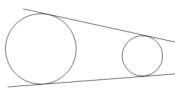Two internal tangents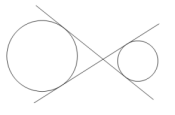If the two circles touch at just one point, there are three possible tangent lines that are common to both: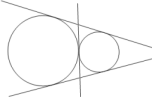If the two circles touch at just one point, with one inside the other, there is just one line that is a tangent to both: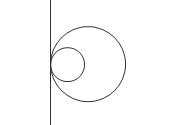If the circles overlap – i.e. intersect at two points, there are two tangents that are common to both: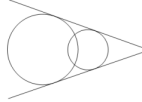If the circles lie one inside the other, there are no tangents that are common to both. A tangent to the inner circle would be a secant of the outer circle.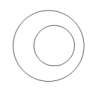### Solved Examples of Tangent to a Circle

Question: Determine the equation of the tangent to the circle:

$$\begin{array}{l}x^{2}+y^{2}-2y+6x-7=0\;at\;the\;point\;F(-2:5)\end{array}$$

Solution:

Write the equation of the circle in the form:

$$\begin{array}{l}\left(x-a\right)^{2}+\left(y-b\right)^{2}+r^{2}\end{array}$$

Use the method of completing the square:

$$\begin{array}{l}x^{2}+y^{2}-2y+6x-7=0\end{array}$$

$$\begin{array}{l}x^{2}+6x+y^{2}-2y=7\end{array}$$

$$\begin{array}{l}\left(x^{2}+6x+9\right)-9+\left(y^{2}-2y+1\right)-1=7\end{array}$$

$$\begin{array}{l}\left(x+3\right)^{2}+\left(y-1\right)^{2}=17\end{array}$$

Draw a sketch

The centre of the circle is (−3;1) and the radius is

$$\begin{array}{l}\sqrt{17}\end{array}$$
units.$$\begin{array}{l}m_{CF}=\frac{y_{2}-y_{1}}{x_{2}-x_{1}}\end{array}$$

$$\begin{array}{l}=\frac{5-1}{-2+3}=4\end{array}$$

Determine the gradient of the tangent

Let the gradient of the tangent line be m.

$$\begin{array}{l}m_{CF}\times m=-1\end{array}$$

$$\begin{array}{l}4\times m=-1\end{array}$$

Therefore,

$$\begin{array}{l} m=-\frac{1}{4}\end{array}$$

Determine the equation of the tangent to the circle

Write down the gradient-point form of a straight line equation and substitute

$$\begin{array}{l}m=-\frac{1}{4}\;and\;F(-2:5)\end{array}$$

So,

$$\begin{array}{l}y-y_{1}=m\left(x-x_{1}\right)\end{array}$$

$$\begin{array}{l}y-y_{1}=-\frac{1}{4}\left(x-x_{1}\right)\end{array}$$

$$\begin{array}{l}Substitute\;F\left(-2:5\right):\;y-5=-\frac{1}{4}\left(x-\left(-2\right)\right)\end{array}$$

$$\begin{array}{l}y-5=-\frac{1}{4}\left (x+2 \right )\end{array}$$

$$\begin{array}{l}y=-\frac{1}{4}x-\frac{1}{2}+5\end{array}$$

$$\begin{array}{l}=-\frac{1}{4}x+\frac{9}{2}\end{array}$$

$$\begin{array}{l}F\;is\;y=-\frac{1}{4}x+\frac{9}{2}\end{array}$$